Goertzel AlgorithmNext: NDFE Algorithm Up: Performance Evaluation and Real-Time Previous: Dual-Tone Multiple Frequencies

# Goertzel Algorithm

The Goertzel algorithm is more efficient than the Fast Fourier Transform in computing an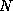-point DFT if less than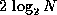DFT coefficients are required . In DTMF detection, we only need 8 of, for example, 205 DFT coefficients to detect the first harmonics of the 8 possible tones, and then apply decision logic to choose the strongest touch tone. Since DTMF signals do not have second harmonics, we could compute another 8 DFT coefficients to compute the second harmonics to detect the presence of speech .

The Goertzel algorithm computes theth DFT coefficient of the input signalusing a second-order filter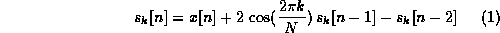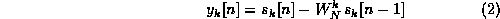where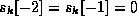. Theth DFT coefficient is produced after the filter has processedsamples: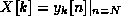. The key in an implementation is to run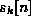forsamples and then evaluate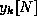. The computation fortakes one add (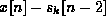) and one multiply-accumulate per sample. In DTMF detection, we are only concerned with the power of theth coefficient,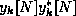: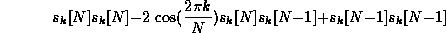The value ofmust be shorter than the samples in half of a DTMF signaling interval (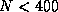), be large enough for good frequency resolution (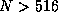), and meet the relative error specification 2(a). We used a conventional value of= 205  because it is roughly half of 400 samples. Decision logic can be added to give a valid DTMF signal if the same two DTMF tones are detected in a row to add robustness against noise.

Table 1: Chosenvalues to minimize the error in.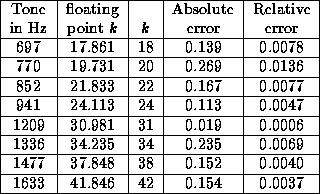Brian L. Evans, 211-105 Cory Hall, Berkeley, CA 94720-1772